## ==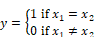Block Category: Boolean

Inputs: Real, complex, or fixed-point scalars, or vectors or matrices.

On the input connector tabs, “l” represents the x1 and “r” represents the x2.

Description: The == block is useful for evaluating the Boolean == equality. This block produces an output signal of 1 if and only if input signal x1 is identically equal to input signal x2; otherwise, the output is zero.

As with programming in any language, it is generally not a good idea to perform Boolean equality comparisons involving floating point variables and non-integer constants. These types of comparisons should be converted to Boolean inequality comparisons. (For example, {If position is equal to π, then…}) should be converted to {If position is greater than or equal to < π rounded off>, then…}.) The reason for this is because a floating-point variable, such as position, is rarely exactly equal to a non-zero non-integer value, particularly if it is obtained by solving one or more equations.

Right-click the == block to assign a different function to the block.

#### Examples

1. Comparing constants

Consider a variable y such that:

If x = 0.5 then y = cos( 2t ); else y = 0

where t is simulation time. Let x be a step function of amplitude 0.5, delayed by 3 sec. This is usually represented as 0.5 u(t - 3). This system can be realized as: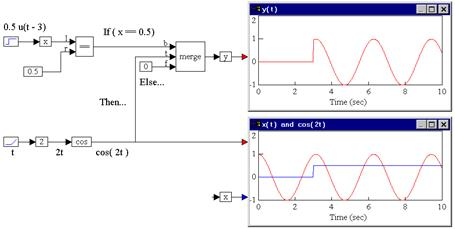Until the onset of the step input at t = 3 sec, the Boolean equality x == 0.5 evaluates to false, and y takes on a value of 0. At t = 3 sec, the Boolean equality evaluates to true, and remains true for the duration of the simulation. Consequently, from this point onwards, y takes on the value of cos(2t). The lower plot block is used to monitor the outputs of the cos block and the variable x.

2. Comparing a floating-point variable with a non-integer constant

In a collision detection problem, if position x of a mass in motion is equal to π, then a collision is assumed to have occurred with an immovable wall that is located at x = π. Furthermore, the position of the mass is assumed to be given by the solution of the following first order differential equation: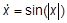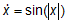The initial condition is assumed to be x(0) = 3.0. It is tempting to realize this system as: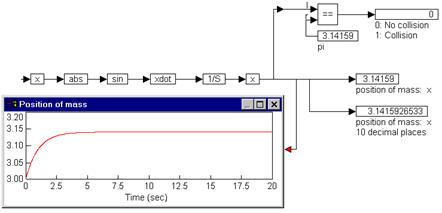From the result shown in the plot block, at around t = 7 sec, the mass arrives at the boundary located at π. However, the collision detection logic, using an == block that compares x with a constant value of π, never detects the collision. This is because the final mass position, as obtained from the output of the integrator, is 3.141592653, which is not equal to 3.14159.

It is clear from the plot block, that for all practical purposes, the mass collided with the wall around t = 7 sec. To capture this reality in the simulation, convert the Boolean equality comparison:

If x = 3.14159 then…

to a Boolean inequality comparison:

If x ≥ 3.1415 then…

After reducing the const block to four decimal places with no round-off, the system can be realized as: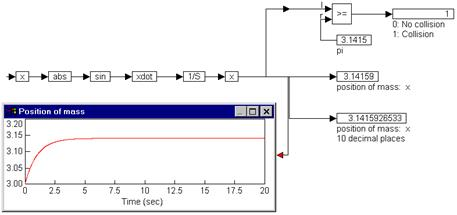Except for replacing the == block with the >= block, this diagram is like the previous one. In this case, the collision detection logic detects a collision around  t = 8 sec. Obviously, the time at which the collision is detected depends on the number of decimal places retained for the π approximation.